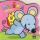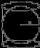# Body volume - math word problems

#### Number of problems found: 575

• Cuboid - ratiosThe sizes of the edges of the cuboid are in the ratio 2: 3: 5. The smallest wall have area 54 cm2. Calculate the surface area and volume of this cuboid.
• Granite cubeWhat is the weight in kg granite cube with an edge of 0.5 m if 1dm3 of granite weight 2600 g?
• FlowerbedThe flowerbed has a length 3500mm and a width 1400mm. How many foil is needed to covers the flowerbed? How many m2 of foil was consumed for its production (add 10% of the material to the joint and waste)? How many liters of air is inside the enclosure? (FThe regular quadrangular prism has a base edge a = 7.1 cm and side edge = 18.2 cm long. Calculate its volume and surface area.
• Square prismCalculate the volume of a foursided prism 2 dm high, the base is a trapezoid with bases 12 cm, 6 cm, height of 4 cm and 5 cm long arms.
• Mouse HryzkaMouse Hryzka found 27 identical cubes of cheese. She first put in a large cube out of them and then waited for a while before the cheese cubes stuck together. Then from every wall of the big cube she will eats the middle cube. Then she also eats the cube
• Rectangle poolDetermine dimensions of open pool with a square bottom with a capacity 32 m3 to have painted/bricked walls with least amount of material.
• Cuboid heightWhat is the height of the cuboid if the edges of its base are 15 cm and 4 cm long and its volume is 420 cm cubic?
• Cone area and sideCalculate the surface area and volume of a rotating cone with a height of 1.25 dm and 17,8dm side.
• Water flow 2How many litres of water will flow in 7 minutes from a cylindrical pipe 1 cm in diameter, if the water flows at a speed of 30 km per hour
• Equilateral cylinderA sphere is inserted into the rotating equilateral cylinder (touching the bases and the shell). Prove that the cylinder has both a volume and a surface half larger than an inscribed sphere.
• Cuboid - volume and areasThe cuboid has a volume of 250 cm3, a surface of 250 cm2 and one side 5 cm long. How do I calculate the remaining sides?
• Triangular prismCalculate the volume and surface of the triangular prism ABCDEF with base of a isosceles triangle. Base's height is 16 cm, leg 10 cm, base height vc = 6 cm. The prism height is 9 cm.
• WinchThe steel rope has a diameter of 6mm and a length of 20m. We are winding on drum width 60mm, starting diameter 50mm. What is the final diameter after winding?
• The coilHow many ropes (the diameter 8 mm) fit on the coil (threads are wrapped close together) The coil has dimension: the inner diameter 400mm, the outside diameter 800mm and the length of the coil is 470mm
• The tankThe tank has 1320 liters of water. The tank has the shape of a prism, its base is an rectangle with sides a = 0,6 m and b = 1,5 m. How high does the water level reach in the tank?
• RainfallThe annual average rainfall in India was in Cherrapunji in the year 1981 26 461 mm. How many hectoliters of water fell on 1 m2? Would fit this amount of water into a cube of three meters?
• Cube, cuboid, and sphereVolumes of a cube and a cuboid are in ratio 3: 2. Volumes of sphere and cuboid are in ratio 1: 3. In what ratio are the volumes of cube, cuboid, and sphere?
• Triangular prismBase of perpendicular triangular prism is a right triangle with leg length 5 cm. Content area of the largest side wall of its surface is 130 cm² and the height of the body is 10 cm. Calculate its volume.
• Cuboid and eq2Calculate the volume of cuboid with square base and height 6 cm if the surface area is 48 cm2.

Do you have an interesting mathematical word problem that you can't solve it? Submit a math problem, and we can try to solve it.

We will send a solution to your e-mail address. Solved examples are also published here. Please enter the e-mail correctly and check whether you don't have a full mailbox.

Please do not submit problems from current active competitions such as Mathematical Olympiad, correspondence seminars etc...